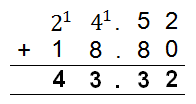Mathematics
Easy

Question

# James works on a 6-hour shift today. He has 2 cartons of items that he needs to deliver today. One is 24.52 kilograms and the other one is 18.8 kilograms. Total weight of cartons James carrying = ________________

## 43.52 kg42.52 kg44.32 kg43.32 kgHint:

## The correct answer is: 43.32 kg

### 24.52 + 18.8 = 24.52 + 19 (rounding to the nearest whole number) = 43.52 - 0.2 (compensation) = 43.32

Number of cartons = 2
Weight of carton 1 = 24.52 kg
Weight of carton 2 = 18.8 kg
Total weight of cartons = weight of carton 1  + weight of carton 2
= 24.52 + 18.8
= 43.32 kgThe correct option is (c) 43.32 kg

### Related Questions to study#### With Turito Foundation.#### Get an Expert Advice From Turito.